# Lab 4A: Data Visualization (Easy)

### Visualizing One Categorical Variable

1. Create a frequency bar chart of ratings.
``````plot(
x = movies\$Rating,
main = "Frequency of Movies by Rating",
xlab = "Rating",
ylab = "Count of Movies")``````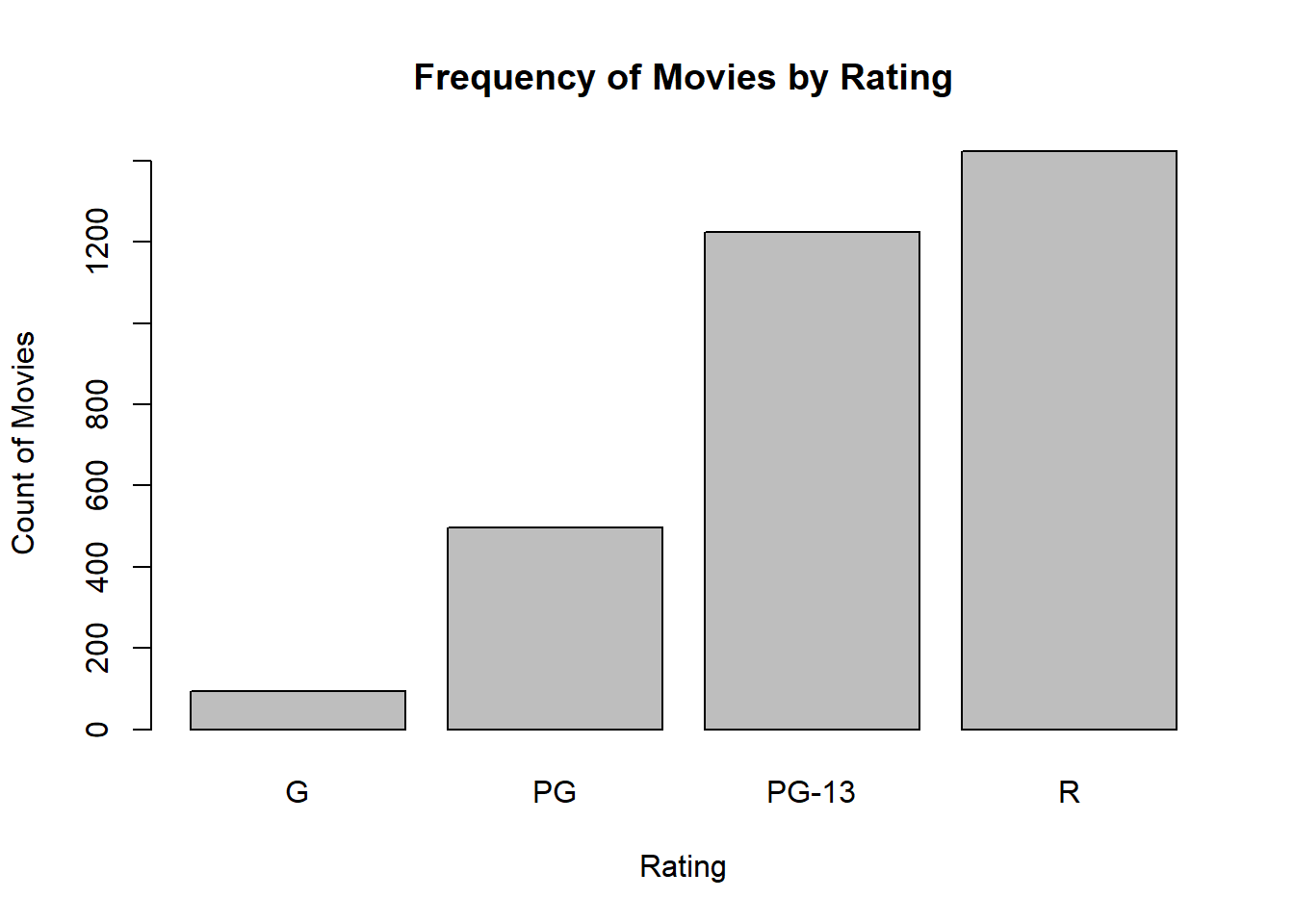1. Create a pie chart of ratings.
``pie(table(movies\$Rating))``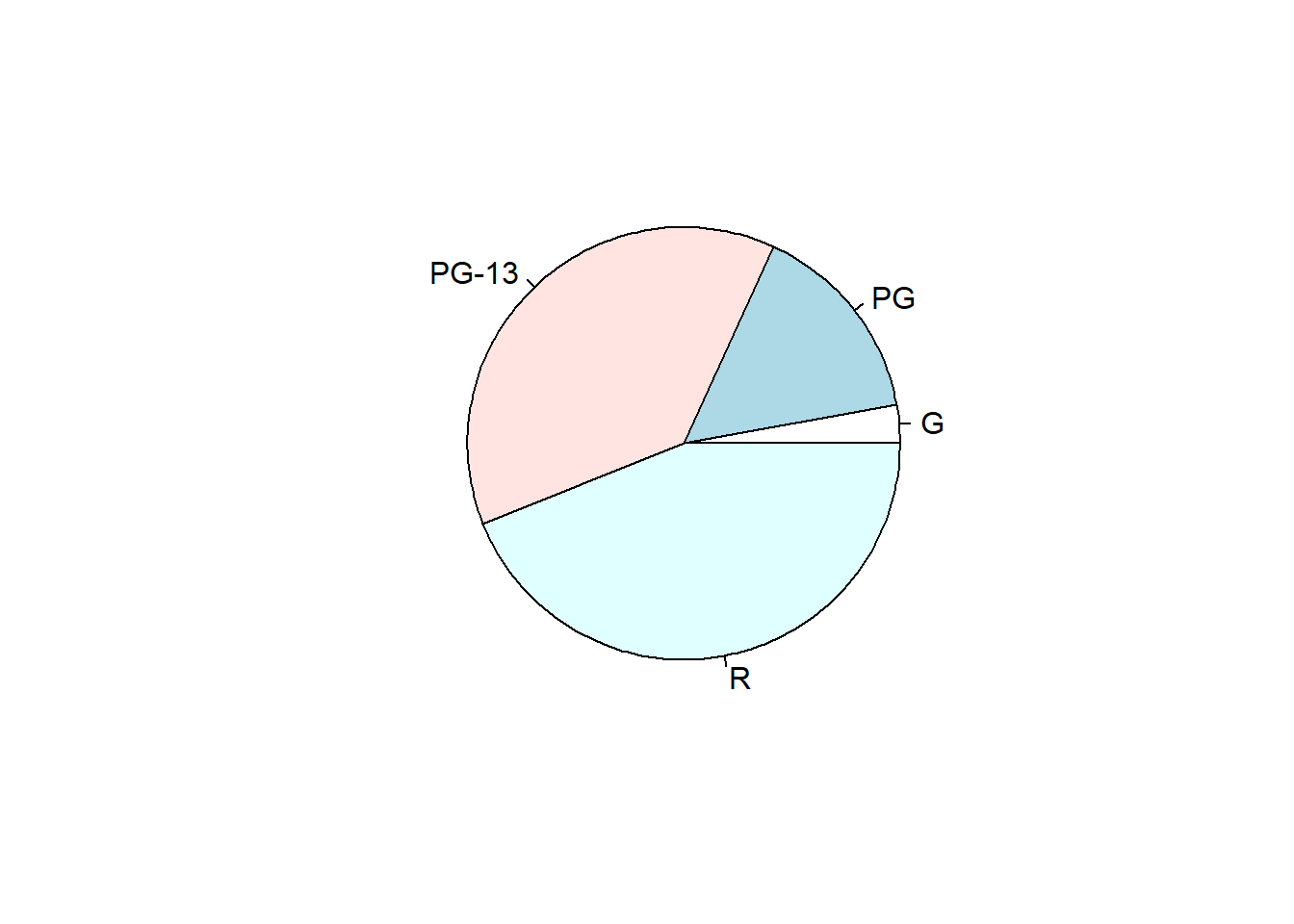### Visualizing One Numeric Variable

1. Create a dot plot of runtime.
``````plot(
x = movies\$Runtime,
y = rep(0, nrow(movies)),
main = "Distribution of Movie Runtime",
xlab = "Runtime (min)",
ylab = "",
yaxt = "n")``````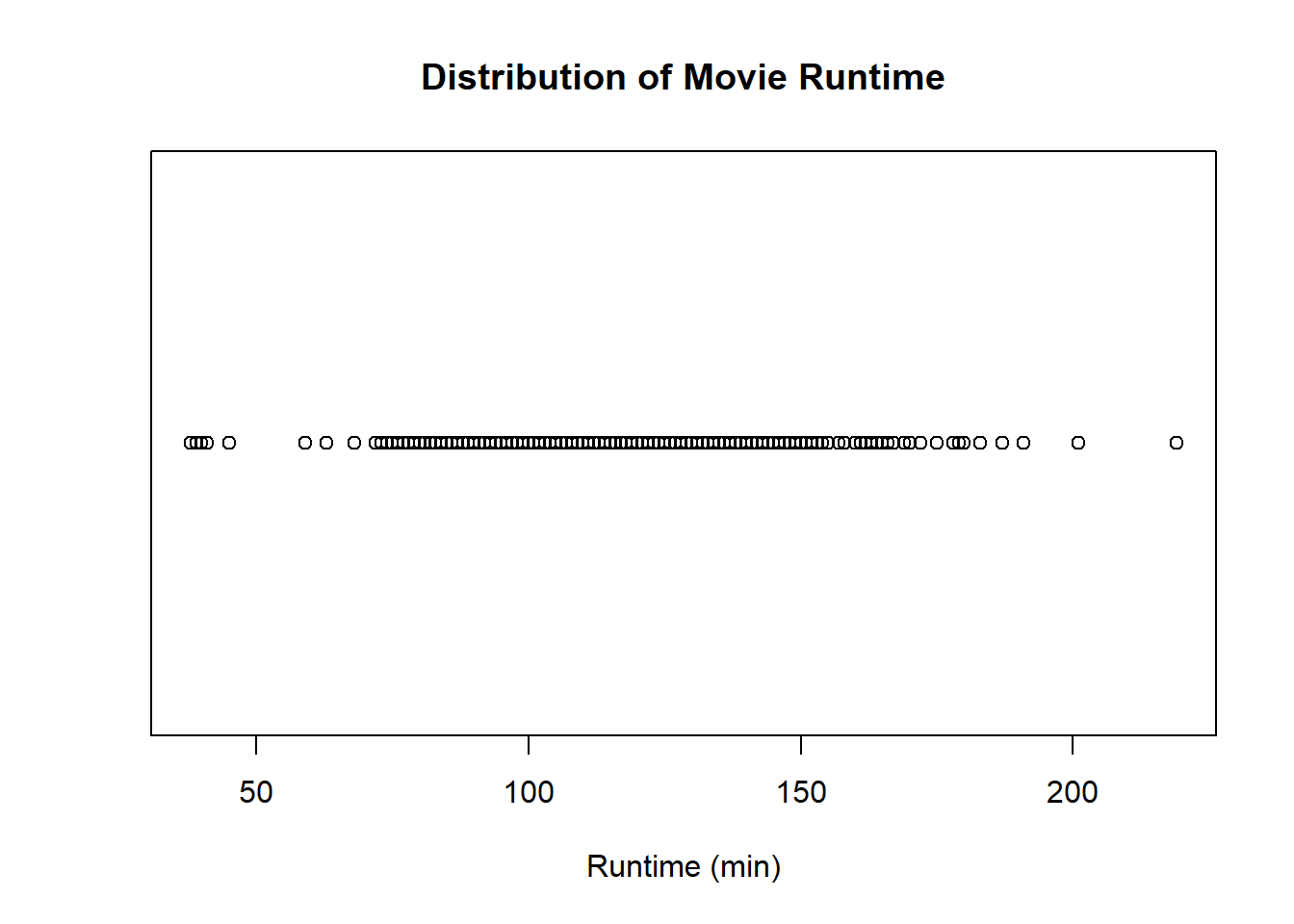1. Create a boxplot of runtime.
``````boxplot(
x = movies\$Runtime,
main = "Distribution of Movie Runtime",
xlab = "Runtime (min)",
horizontal = TRUE)``````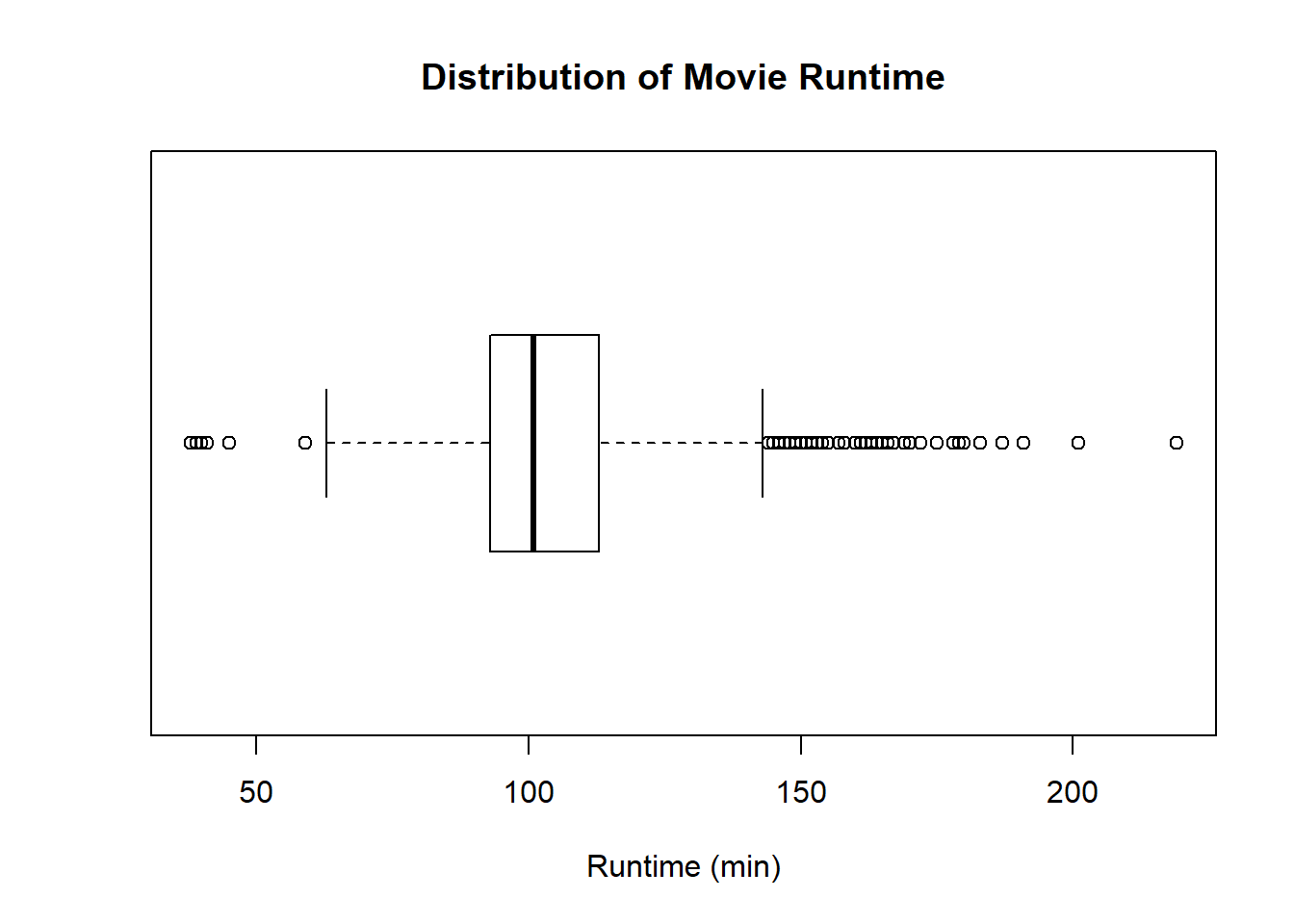1. Create a histogram of runtime.
``````hist(
x = movies\$Runtime,
main = "Distribution of Movie Runtime",
xlab = "Runtime (min)",
ylab = "Frequency")``````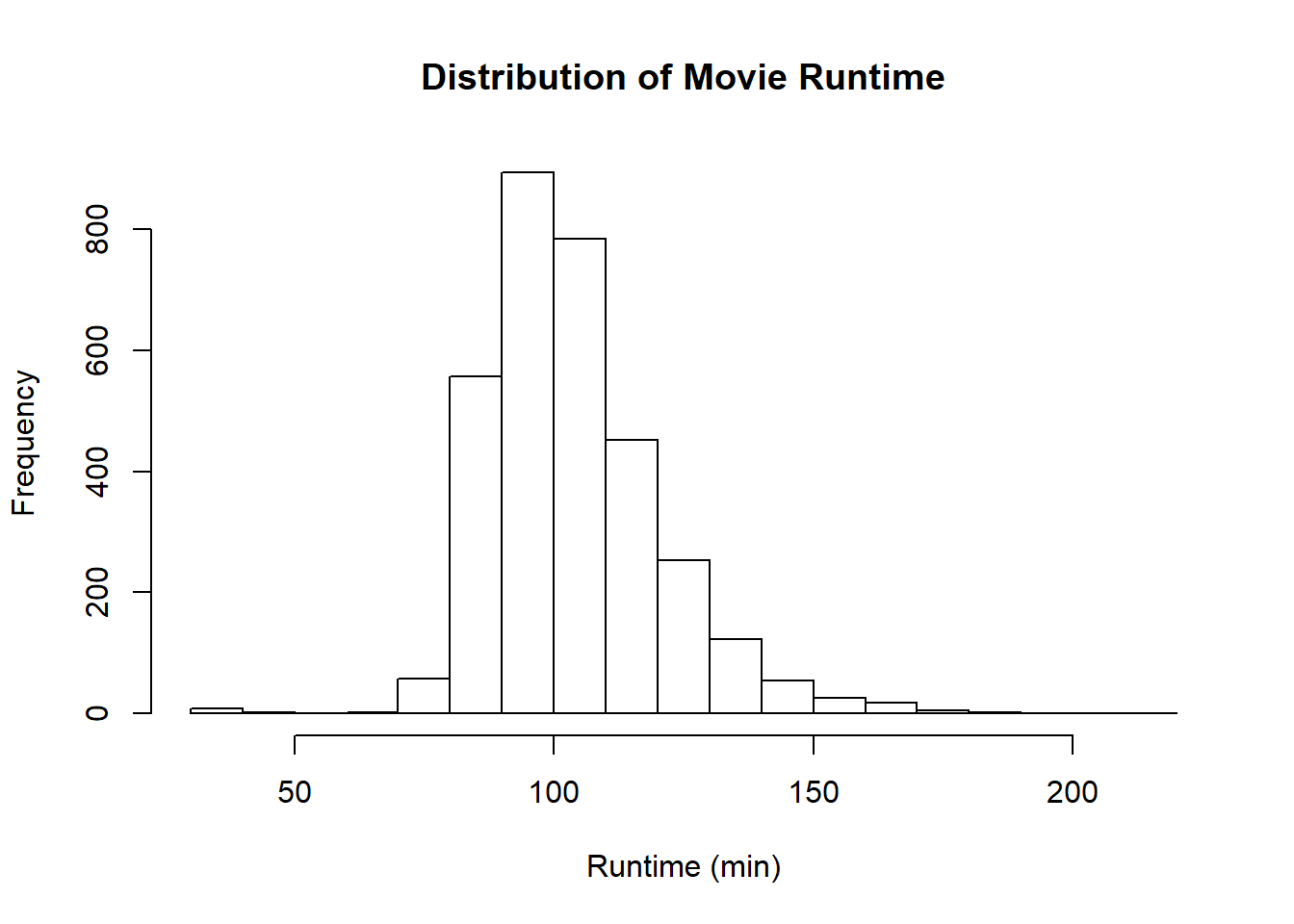1. Create a density plot of runtime.
``````plot(
x = density(movies\$Runtime),
main = "Distribution of Movie Runtime",
xlab = "Runtime (min)",
ylab = "Density")``````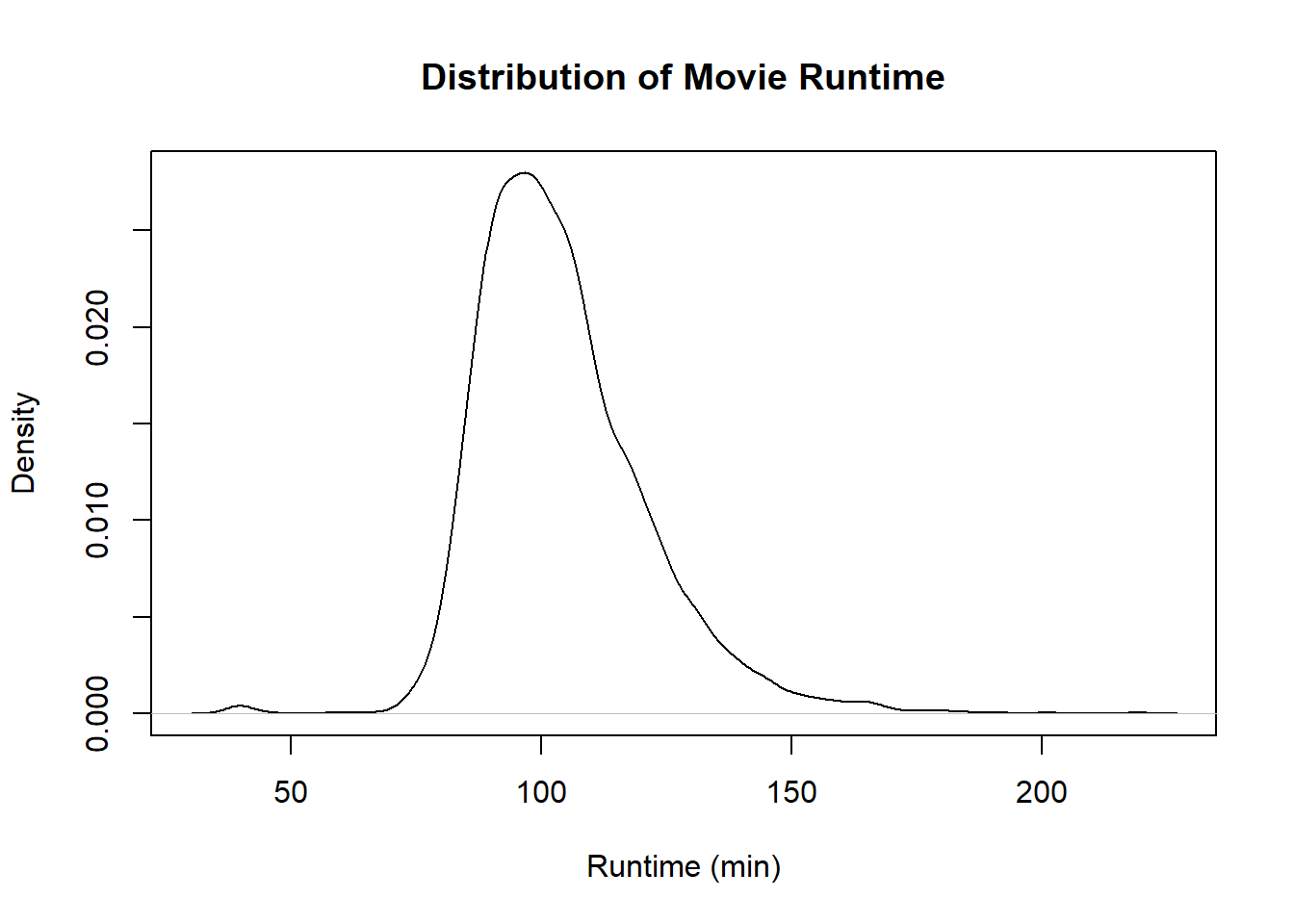### Visualizing Two Categorical Variables

1. Create a spineplot of genre and rating.
``````spineplot(
x = genres\$Genre,
y = genres\$Rating,
main = "Frequency of Movies by Rating and Genre",
xlab = "Genre",
ylab = "Rating")``````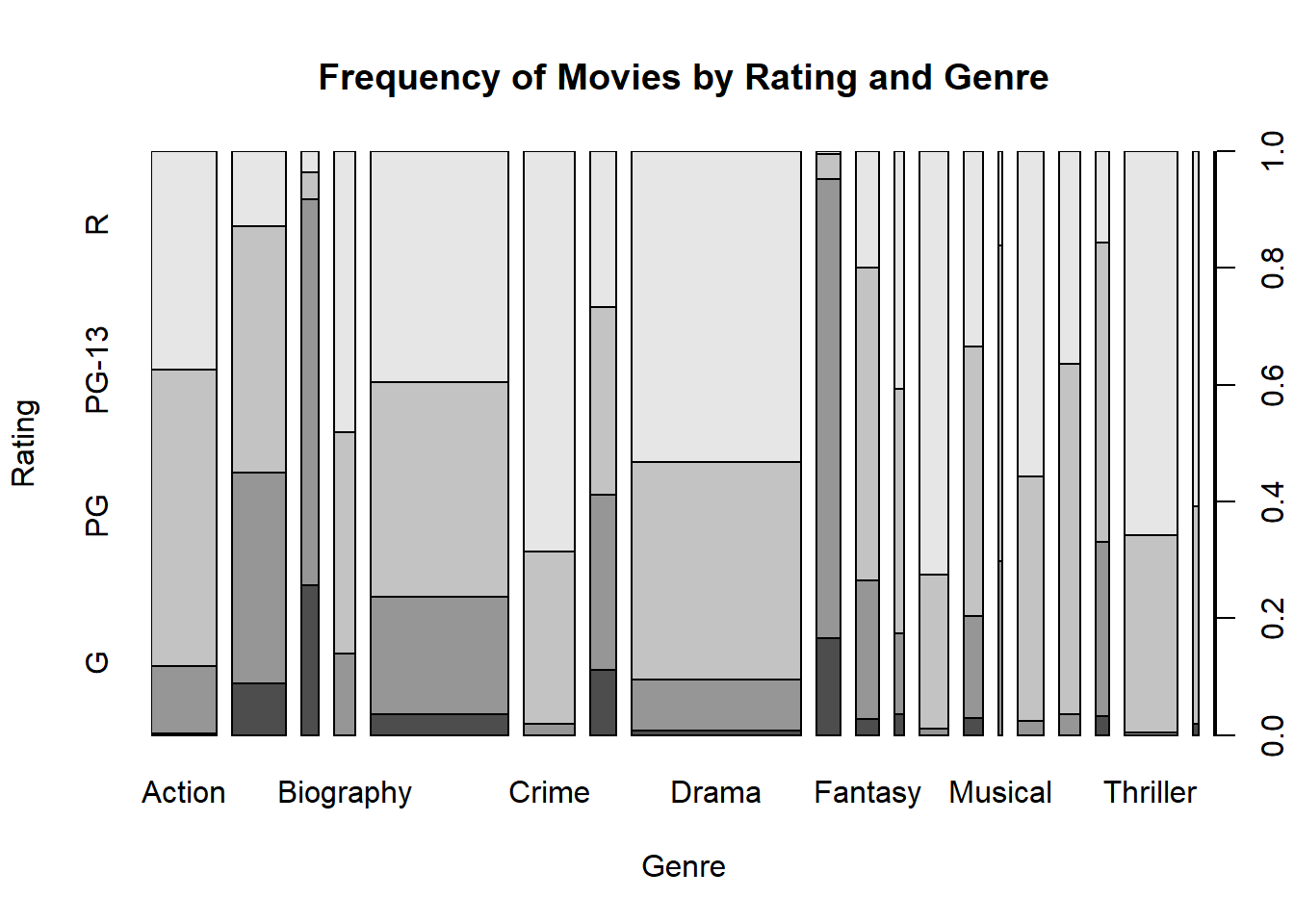1. Create a mosaic plot of genre and rating.
``````mosaicplot(
x = table(
genres\$Genre,
genres\$Rating),
las = 3,
main = "Frequency of Movies by Rating and Genre",
xlab = "Genre",
ylab = "Rating")``````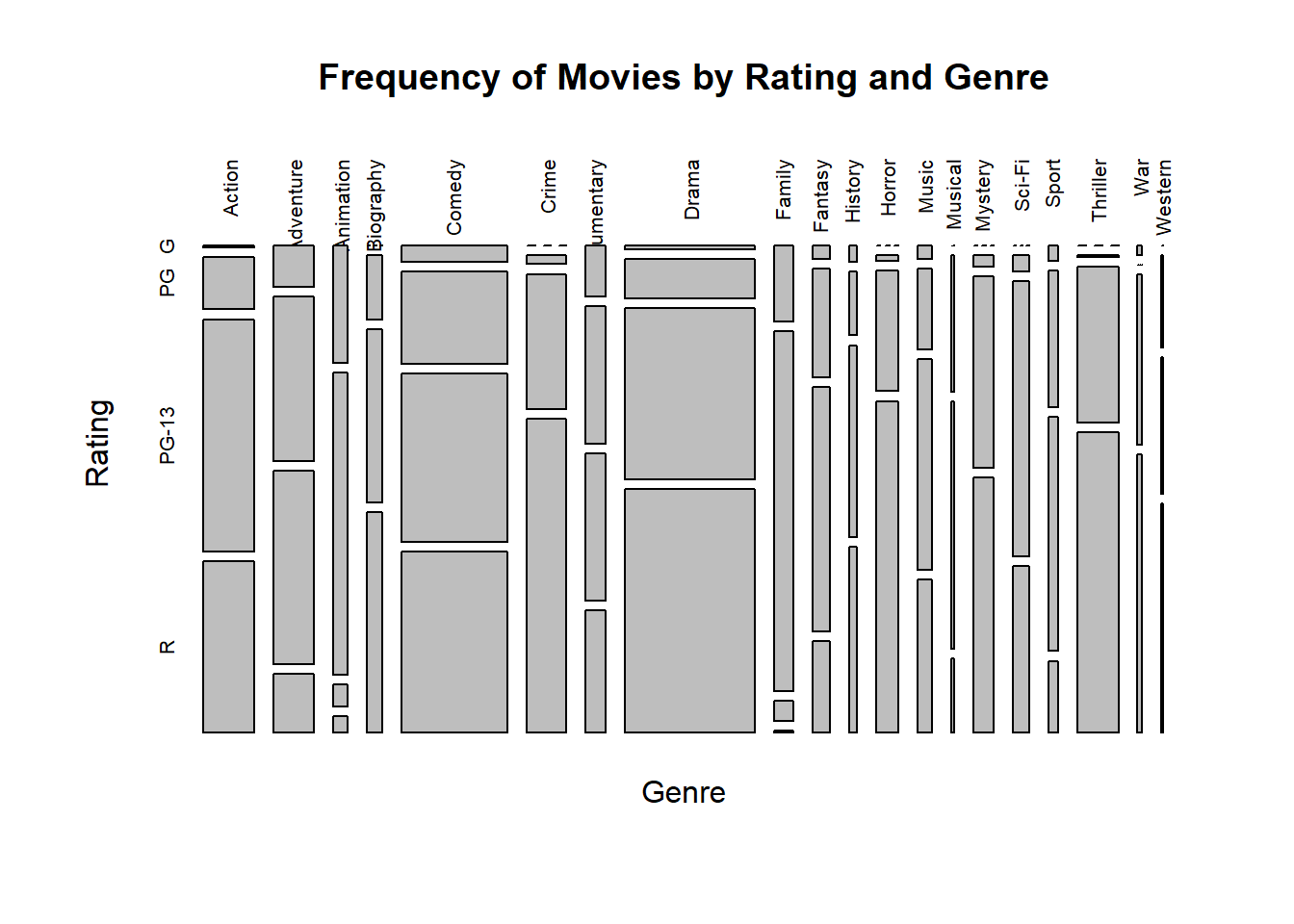### Visualizing Two Numeric Variables

1. Create a scatterplot of runtime and box office.
``````plot(
x = movies\$Runtime,
y = movies\$Box.Office,
main = "Movie Runtime and Box Office Revenue",
xlab = "Runtime (min)",
ylab = "Box Office (\$M)")``````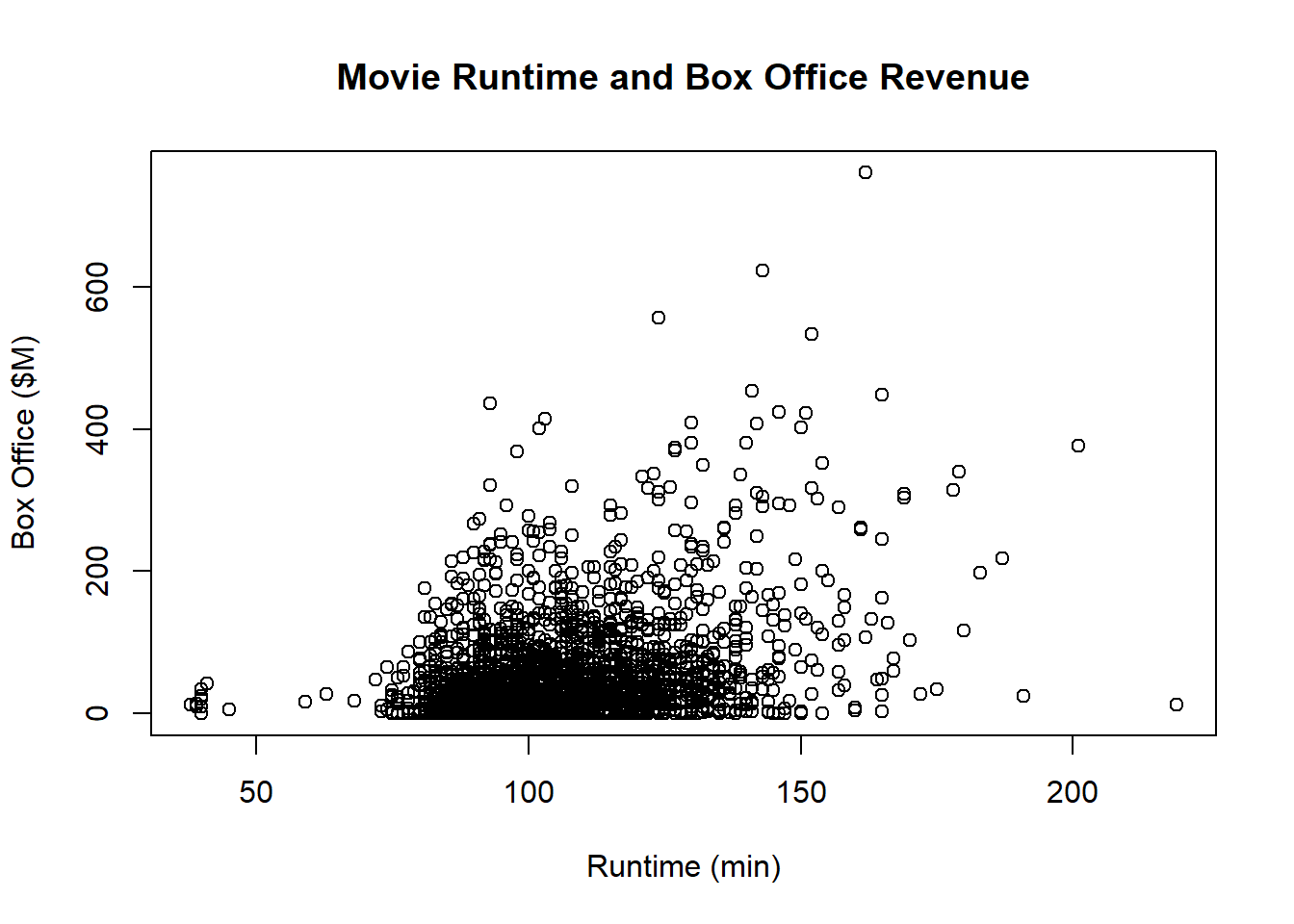1. Create a scatterplot of critic score and box office.
``````plot(
x = movies\$Critic.Score,
y = movies\$Box.Office,
main = "Critic Score and Box Office Revenue",
xlab = "Critic Score (%)",
ylab = "Box Office (\$M)")``````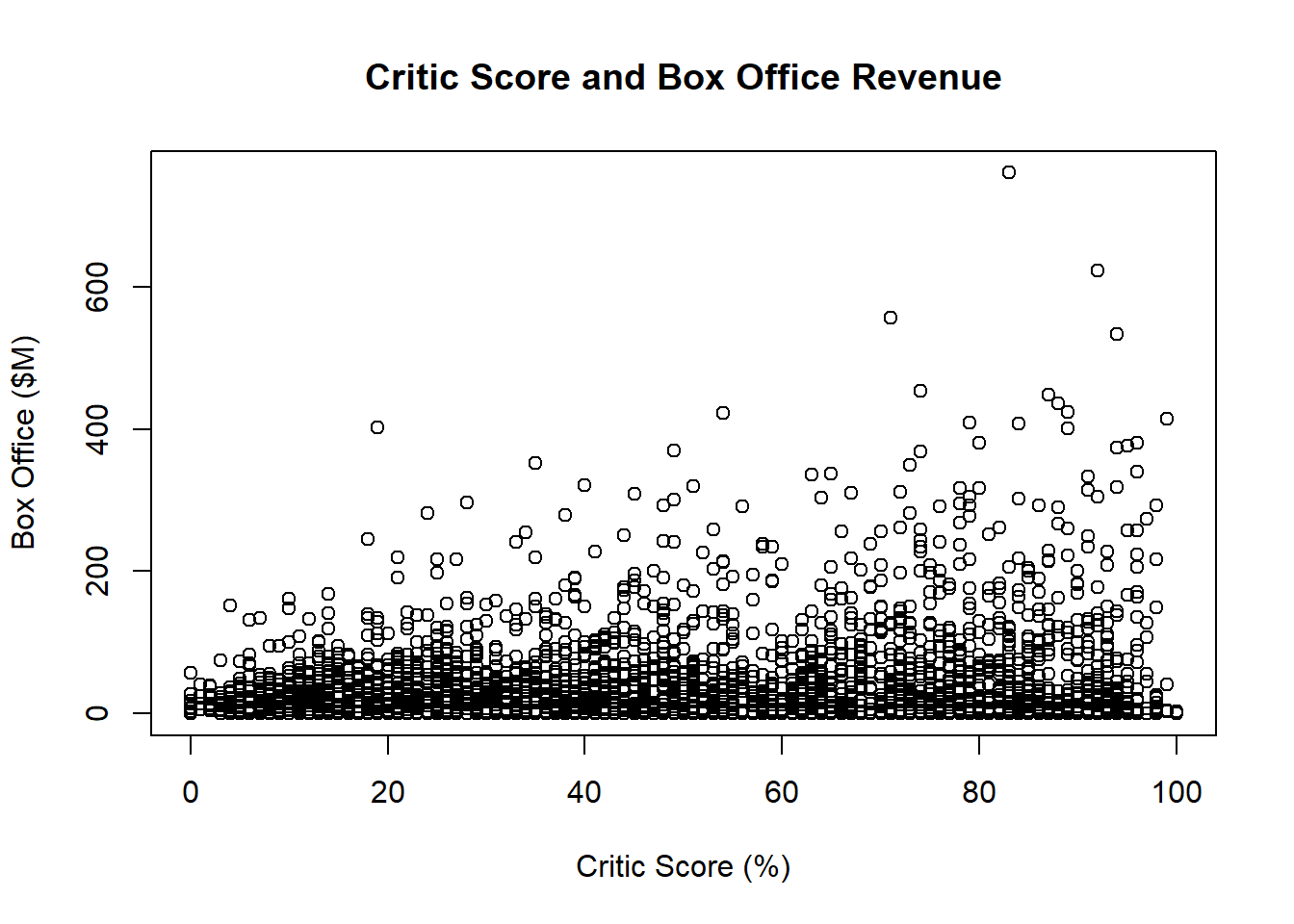1. Plot a line chart of count of movies by year.
``````plot(
x = table(movies\$Year),
type = "l",
main = "Count of Movies by Year",
xlab = "Year",
ylab = "Count of Movies")``````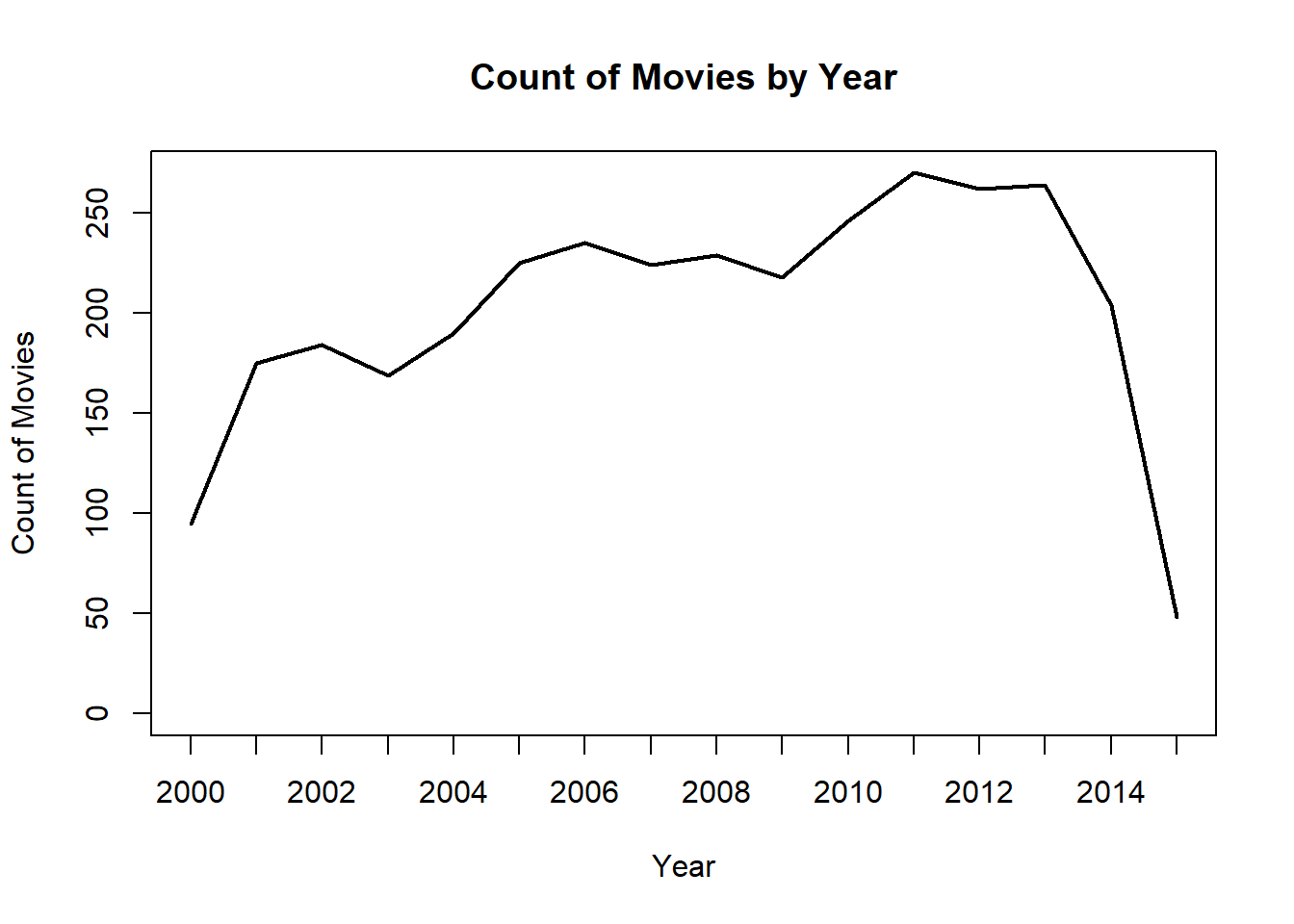### Grouped By a Categorical Variable

1. Create a bar graph of average box office by rating.
``````barplot(
height = tapply(movies\$Box.Office, movies\$Rating, mean),
main = "Average Box Office Revenue by Rating Category",
xlab = "Rating",
ylab = "Box Office (\$M)")``````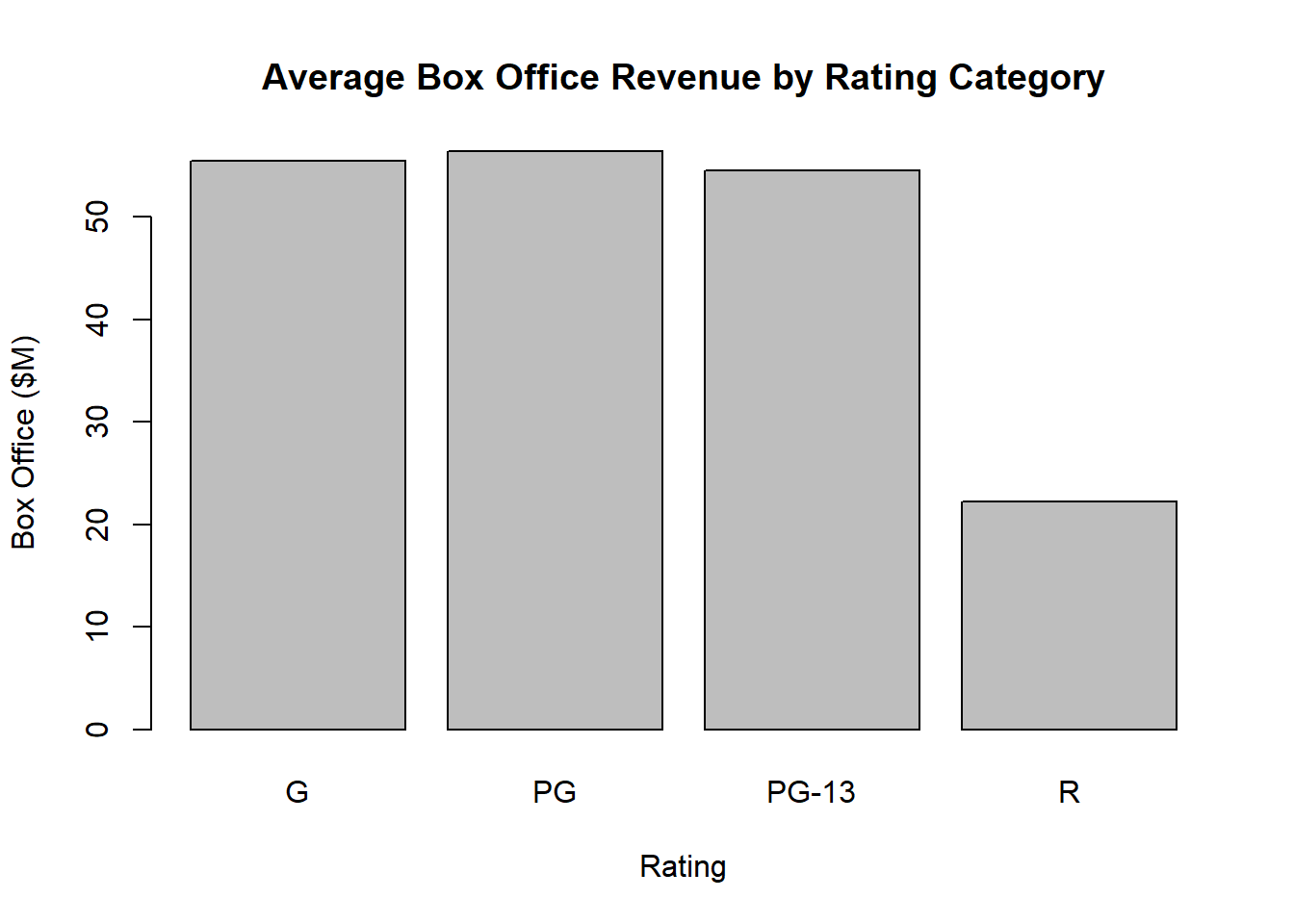1. Create a bar graph of average box office by genre.
``````barplot(
height = tapply(genres\$Box.Office, genres\$Genre, mean),
las = 3,
main = "Average Box Office Revenue by Genre",
ylab = "Box Office (\$M)")``````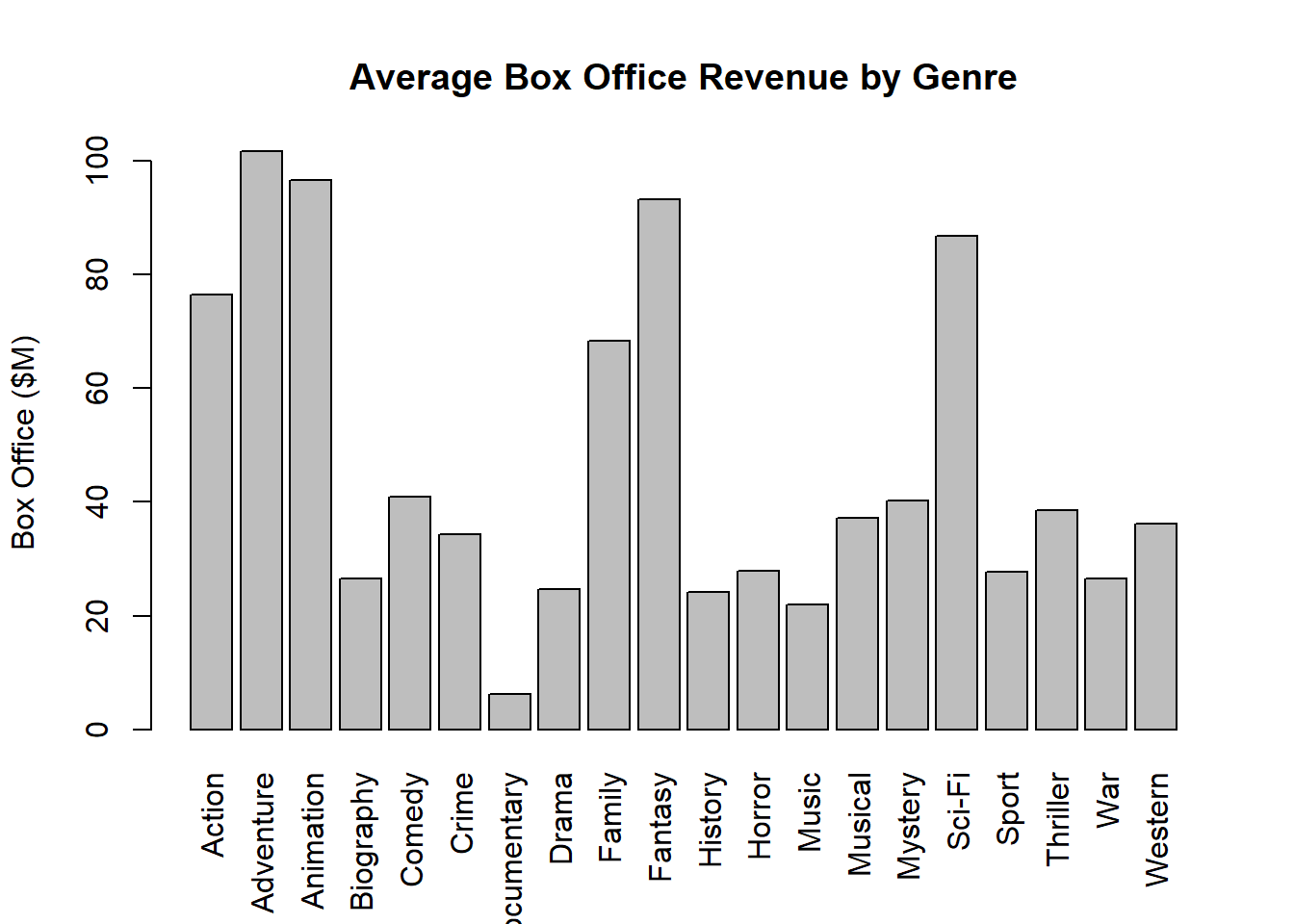### Visualzing Many Variables

1. Create a scatterplot matrix.
``plot(movies)``